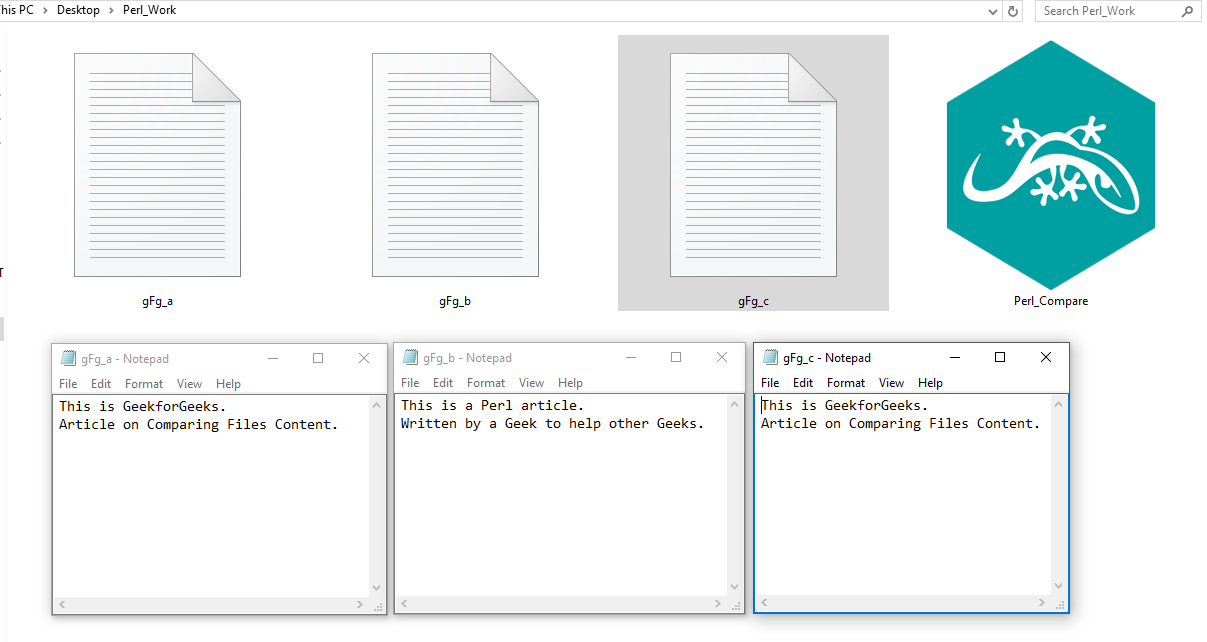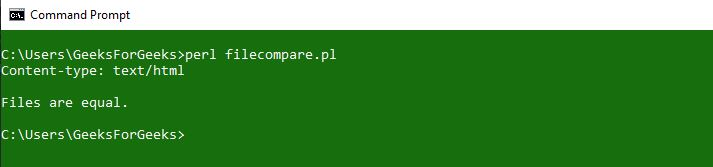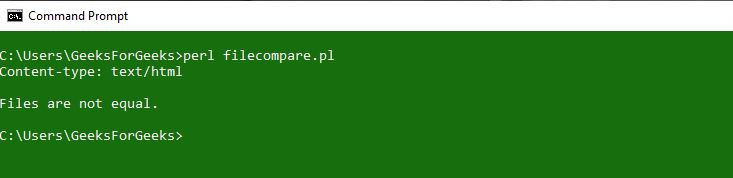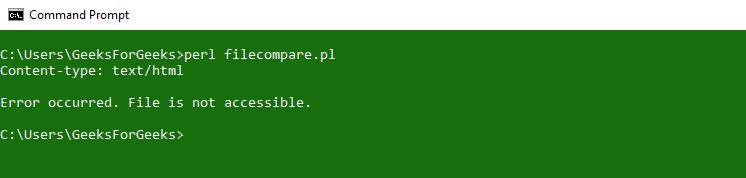# Comparing content of files using Perl

In Perl, we can easily compare the content of two files by using the `File::Compare` module. This module provides a function called `compare`, which helps in comparing the content of two files specified to it as arguments. If the data present in both the files comes out to be same, the function returns 0 as the output, if the data in the passed files is different, the return value is 1 and if there is any error occurred while accessing the specified/passed files, it returns the value as -1.

Syntax:

```use File::Compare;
\$compare = compare('FILE_NAME_1', 'FILE_NAME_2');
```

Note:

• Same Content: Return Value 
• Different Content: Return Value 
• Error in Accessing Files: Return Value [-1]
• Example:

Files Present in the Folder.When the content of the Files is same:

 `#!/usr/bin/perl ` `print` `"Content-type: text/html\n\n"``; ` ` `  `# Module to use compare function ` `use` `File::Compare; ` ` `  `# compare function to access the passed files. ` `\$compare` `= compare(``"gfg_a.txt"``, ``"gfg_c.txt"``); ` ` `  `# checking if the files are same ` `if` `(``\$compare` `== 0) ` `{ ` `    ``print` `"Files are equal. \n"``; ` `} ` ` `  `# checking if the files are different ` `elsif` `(``\$compare` `== 1) ` `{ ` `    ``print` `"Files are not equal. \n"``; ` `} ` ` `  `# checking if the file is not accessible ` `elsif``(``\$compare` `== -1) ` `{ ` `    ``print` `"Error Occured. \n"``; ` `} ` ` `  `exit``; `

Output:When the content of the Files is different:

 `#!/usr/bin/perl ` `print` `"Content-type: text/html\n\n"``; ` ` `  `# Module to use compare function ` `use` `File::Compare; ` ` `  `# compare function to access the passed files. ` `\$compare` `= compare(``"gfg_a.txt"``, ``"gfg_b.txt"``); ` ` `  `# checking if the files are same ` `if` `(``\$compare` `== 0) ` `{ ` `    ``print` `"Files are equal. \n"``; ` `} ` ` `  `# checking if the files are different ` `elsif` `(``\$compare` `== 1) ` `{ ` `    ``print` `"Files are not equal. \n"``; ` `} ` ` `  `# checking if the file is not accessible ` `elsif``(``\$compare` `== -1) ` `{ ` `    ``print` `"Error Occured. \n"``; ` `} ` ` `  `exit``; `

Output:When the file is not accessible:

 `#!/usr/bin/perl ` `print` `"Content-type: text/html\n\n"``; ` ` `  `# Module to use compare function ` `use` `File::Compare; ` ` `  `# compare function to access the passed files. ` `\$compare` `= compare(``"gfg_a.txt"``, ``"gfg_d.txt"``); ` ` `  `# checking if the files are same ` `if` `(``\$compare` `== 0) ` `{ ` `    ``print` `"Files are equal. \n"``; ` `} ` ` `  `# checking if the files are different ` `elsif` `(``\$compare` `== 1) ` `{ ` `    ``print` `"Files are not equal. \n"``; ` `} ` ` `  `# checking if the file is not accessible ` `elsif``(``\$compare` `== -1) ` `{ ` `    ``print` `"Error occurred. The file is not accessible. \n"``; ` `} ` ` `  `exit``; `

Output:My Personal Notes arrow_drop_upCheck out this Author's contributed articles.

If you like GeeksforGeeks and would like to contribute, you can also write an article using contribute.geeksforgeeks.org or mail your article to contribute@geeksforgeeks.org. See your article appearing on the GeeksforGeeks main page and help other Geeks.

Please Improve this article if you find anything incorrect by clicking on the "Improve Article" button below.

Article Tags :
Practice Tags :

Be the First to upvote.

Please write to us at contribute@geeksforgeeks.org to report any issue with the above content.